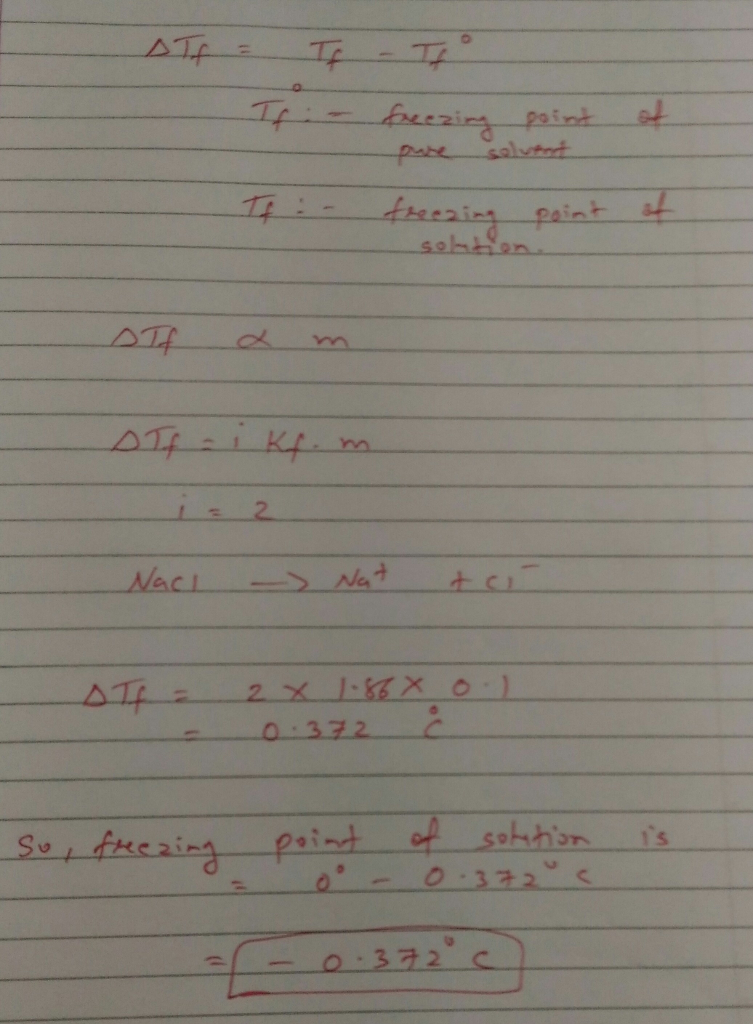# Homework Solution: What is the normal freezing point of 0.100 m aqueous NaCl? Assume that NaCl…

What is the normal freezing point of 0.100 m aqueous NaCl? Assume that NaCl dissociates completely. (K_f for water is 1.86 degree C/m) a) 0.372 degree C b) 0.0 degree C c) -0.186 degree C d) -0.372 degree C